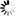DOI: 10.5176/2251-1938_ORS16.20

Authors: G.Nithish Kumar and Debashis Dutta

Abstract:

This paper presents a rough interval approach to determine the preferred compromise solution for fuzzy fixed charge solid transportation problem. The fixed charge solid transportation problem is an extension of classical transportation problem in which a fixed profit is incurred, independent of the amount transported, along with a variable profit that is proportional to the amount shipped. The fixed charge solid transportation has two kinds of profits: direct profit and fixed charge profit. The proposed problem considers fuzzy coefficients for the direct profit, fixed charge and constraints with rough interval is modeled and then solved. Here we construct two solid transportation problems with interval coefficients considering the lower approximation and the upper approximation of the rough intervals. Moreover from these two solid transportation problems four different classical solid transportation problems are constituted and solved using Lingo 13.0 software. Expected values of the fuzzy objective functions (direct profit, fixed charge profit) are considered to derive the crisp values. The concept of the completely satisfactory solution and rather satisfactory solutions, surely optimal range, possibly optimal range and rough optimal range are discussed. Numerical example is presented using the above proposed methodology. Concluding remarks are given in the last section.

Keywords: Fixed charge; Solid transportation problem; Fuzzy sets; Expected value fuzzy programming technique; Rough set theory; Rough interval coefficients.

simplr_role_lock:

Price: \$0.00Updating...